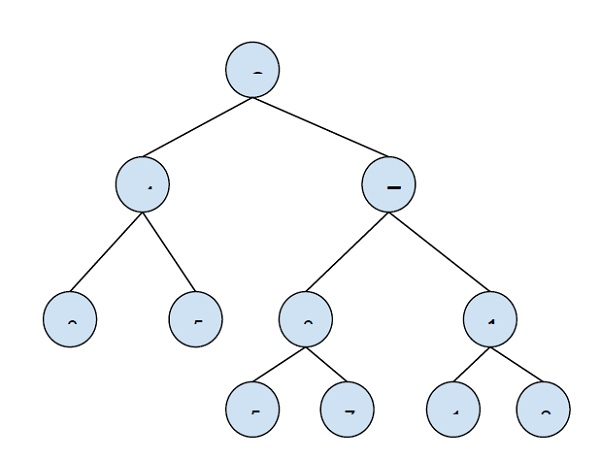# Print all nodes less than a value x in a Min Heap in C++

C++Server Side ProgrammingProgramming

In this problem, we are given a Min Heap and a value x and we have to print all nodes less than x.

Min heap is a special type of binary tree in which every node has a value less than the node value of its child node.

Let’s take an example to understand the problem −X = 45

Output − 2 4 7 10 17 22 33 34

Now, to solve this problem we need to do pre-order traversal of the whole min-heap and print only those values which are less than the given value X. If a value of a node is greater than x then, we will not traverse the child node there value will be greater than x. We will use recursion for doing preorder traversal of the min-heap.

## Example

Program to illustrate the working of our solution

Live Demo

#include <iostream>
using namespace std;
class MinHeap {
int* harr;
int capacity;
int heap_size;
public:
MinHeap(int capacity);
void Heapify(int);
int parent(int i) { return (i - 1) / 2; }
int left(int i) { return (2 * i + 1); }
int right(int i) { return (2 * i + 2); }
void insert(int k);
void printSmallerNodes(int k, int pos);
};
void MinHeap::printSmallerNodes(int x, int pos = 0){
if (pos >= heap_size)
return;
if (harr[pos] >= x) {
return;
}
cout<<harr[pos]<<" ";
printSmallerNodes(x, left(pos));
printSmallerNodes(x, right(pos));
}
MinHeap::MinHeap(int cap) {
heap_size = 0;
capacity = cap;
harr = new int[cap];
}
void MinHeap::insert(int k) {
if (heap_size == capacity) {
cout << "\nOverflow! Size Full\n";
return;
}
heap_size++;
int i = heap_size - 1;
harr[i] = k;
while (i != 0 && harr[parent(i)] > harr[i]) {
swap(harr[i], harr[parent(i)]);
i = parent(i);
}
}
void MinHeap::Heapify(int i) {
int l = left(i);
int r = right(i);
int smallest = i;
if (l < heap_size && harr[l] < harr[i])
smallest = l;
if (r < heap_size && harr[r] < harr[smallest])
smallest = r;
if (smallest != i) {
swap(harr[i], harr[smallest]);
Heapify(smallest);
}
}
int main() {
MinHeap h(50);
h.insert(2);
h.insert(4);
h.insert(7);
h.insert(34);
h.insert(52);
h.insert(33);
h.insert(10);
h.insert(51);
h.insert(75);
h.insert(17);
h.insert(22);
int x = 45;
cout<<"All nodes with value smaller than "<<x<<" are\n";
h.printSmallerNodes(x);
return 0;
}

## Output

All nodes with a value smaller than 45 are 2 4 34 17 22 7 33 10